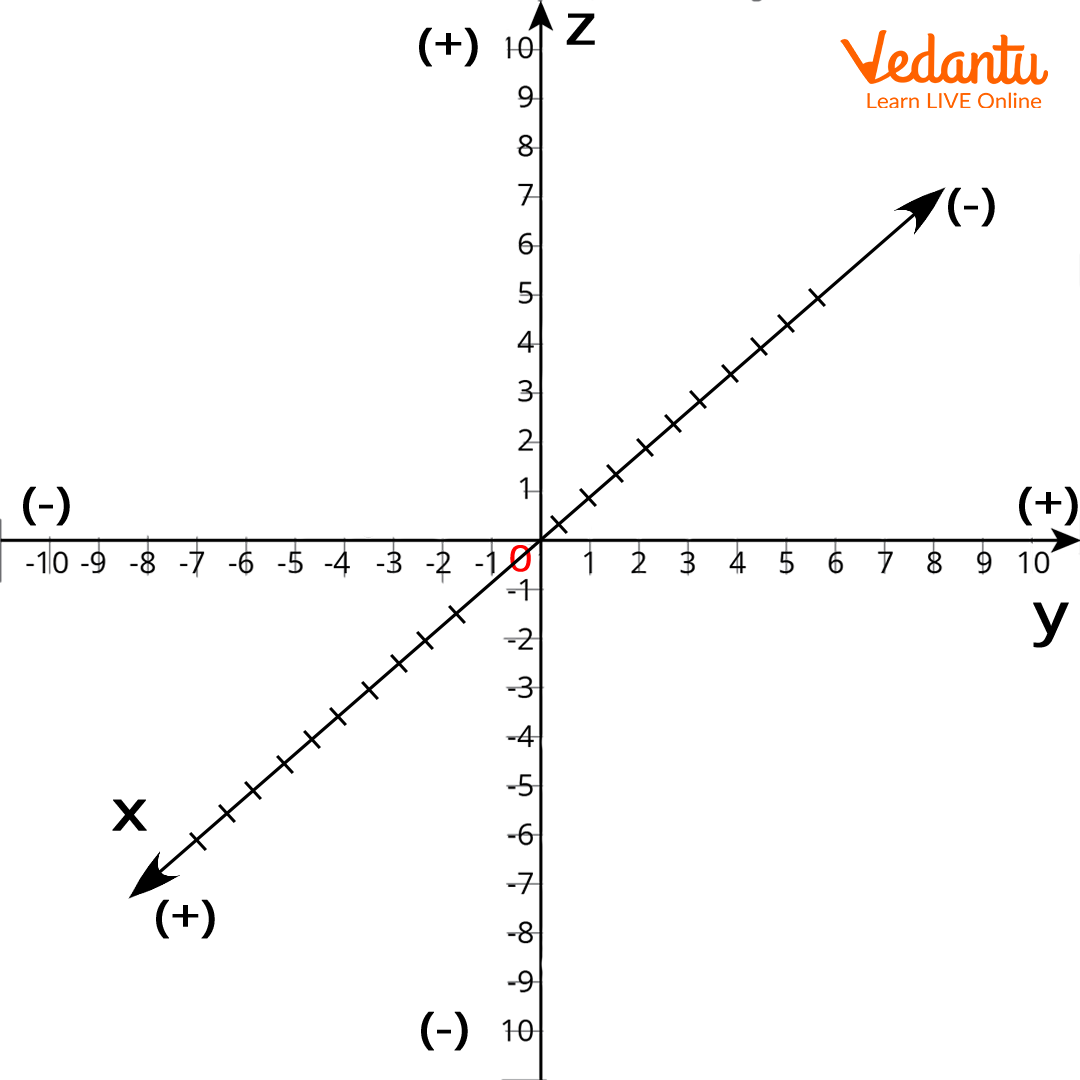Courses
Courses for Kids
Free study material
Free LIVE classes
More## Learn Mathematics Vocabulary with the letter X

The X-letter word in Maths is used quite frequently. It definitely has its importance in various mathematical concepts. In this context, students will be basically learning the Mathematics vocabulary starting with the letter X.

With the help of this learning, the students will develop a new level of vocabulary learning which will help them to understand various concepts starting with the letter X.

## Words with X in Maths Dictionary

X in Maths dictionary are those words that start with the letter X. We are going to explain each concept of the term starting with the initial of X in the mathematics context. So, let us find the math words that start with X.

## The Terms Starting with Letter XX intercept

There are numerous mathematical terms that start with the letter X. Like the letter ‘x’ itself holds value in Maths. Then there is ‘x’ as an axis, ‘x’ as a coordinate, ‘x’ as an intercept, ‘x-y’ as a plane surface, or ‘x-z’ as a plane surface.

As Maths students, it becomes necessary to know about x words in different contexts. If the students want to surf the web and find the different words starting with the letter X that might take time and plus there will be a lack of mathematical knowledge.

So, to avoid such a burden we are presenting a brief concept of the terms starting with X.

The words with their brief explanation are as follows:

1. X

This is a symbol used in the calculation in order to estimate an unknown value in an equation. Like X+5=9

1. X

As a Roman numeral which is the value of 10.

1. X-Axis

This is the axis that is placed horizontally in a coordinate plane or graph.

1. X-Coordinate

This is possibly the first coordinate in an ordered pair like x and y.

1. X- intercept

This is the point on a graph paper where the value of y is zero.

1. X-Y plane

Suppose there is a formation of a plane with two coordinates - x and y. Then that plane will be termed as the ‘x-y’ plane.

1. X-Z plane

Suppose a plane is structured with two co-ordinate axes which are x and z then this will be known as the X-Z plane. Thus, the plane which is formed with two coordinates x and z is called the x-z plane.

Thus, we have discussed 7 mathematical terms starting with the X letter. We will be coming across these x terms in mathematics in different chapters. The students know how these terms are necessary for the students, as it will help them to understand and analyze the concept and calculations better.